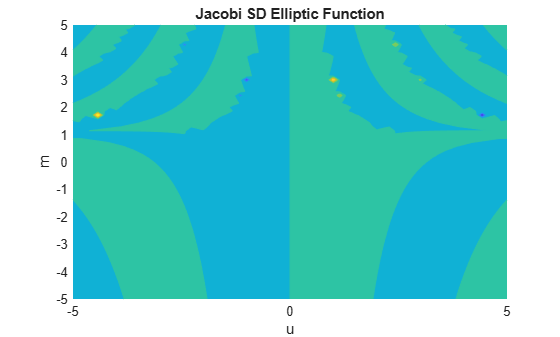# jacobiSD

Jacobi SD elliptic function

## Syntax

``jacobiSD(u,m)``

## Description

example

````jacobiSD(u,m)` returns the Jacobi SD Elliptic Function of `u` and `m`. If `u` or `m` is an array, then `jacobiSD` acts element-wise.```

## Examples

collapse all

`jacobiSD(2,1)`
```ans = 3.6269```

Call `jacobiSD` on array inputs. `jacobiSD` acts element-wise when `u` or `m` is an array.

`jacobiSD([2 1 -3],[1 2 3])`
```ans = 3.6269 2.1629 -126.3078```

Convert numeric input to symbolic form using `sym`, and find the Jacobi SD elliptic function. For symbolic input where `u = 0` or `m = 0` or `1`, `jacobiSD` returns exact symbolic output.

`jacobiSD(sym(2),sym(1))`
```ans = sinh(2)```

Show that for other values of `u` or `m`, `jacobiSD` returns an unevaluated function call.

`jacobiSD(sym(2),sym(3))`
```ans = jacobiSD(2, 3)```

For symbolic variables or expressions, `jacobiSD` returns the unevaluated function call.

```syms x y f = jacobiSD(x,y)```
```f = jacobiSD(x, y)```

Substitute values for the variables by using `subs`, and convert values to double by using `double`.

`f = subs(f, [x y], [3 5])`
```f = jacobiSD(3, 5)```
`fVal = double(f)`
```fVal = 0.0312```

Calculate `f` to higher precision using `vpa`.

`fVal = vpa(f)`
```fVal = 0.031220579864538785956650143970485```

Plot the Jacobi SD elliptic function using `fcontour`. Set `u` on the x-axis and `m` on the y-axis by using the symbolic function `f` with the variable order `(u,m)`. Fill plot contours by setting `Fill` to o`n`.

```syms f(u,m) f(u,m) = jacobiSD(u,m); fcontour(f,'Fill','on') title('Jacobi SD Elliptic Function') xlabel('u') ylabel('m')```## Input Arguments

collapse all

Input, specified as a number, vector, matrix, or multidimensional array, or a symbolic number, variable, vector, matrix, multidimensional array, function, or expression.

Input, specified as a number, vector, matrix, or multidimensional array, or a symbolic number, variable, vector, matrix, multidimensional array, function, or expression.

collapse all

### Jacobi SD Elliptic Function

The Jacobi SD elliptic function is

sd(u,m) = sn(u,m)/dn(u,m)

where sn and dn are the respective Jacobi elliptic functions.

The Jacobi elliptic functions are meromorphic and doubly periodic in their first argument with periods 4K(m) and 4iK'(m), where K is the complete elliptic integral of the first kind, implemented as `ellipticK`.

## Version History

Introduced in R2017b